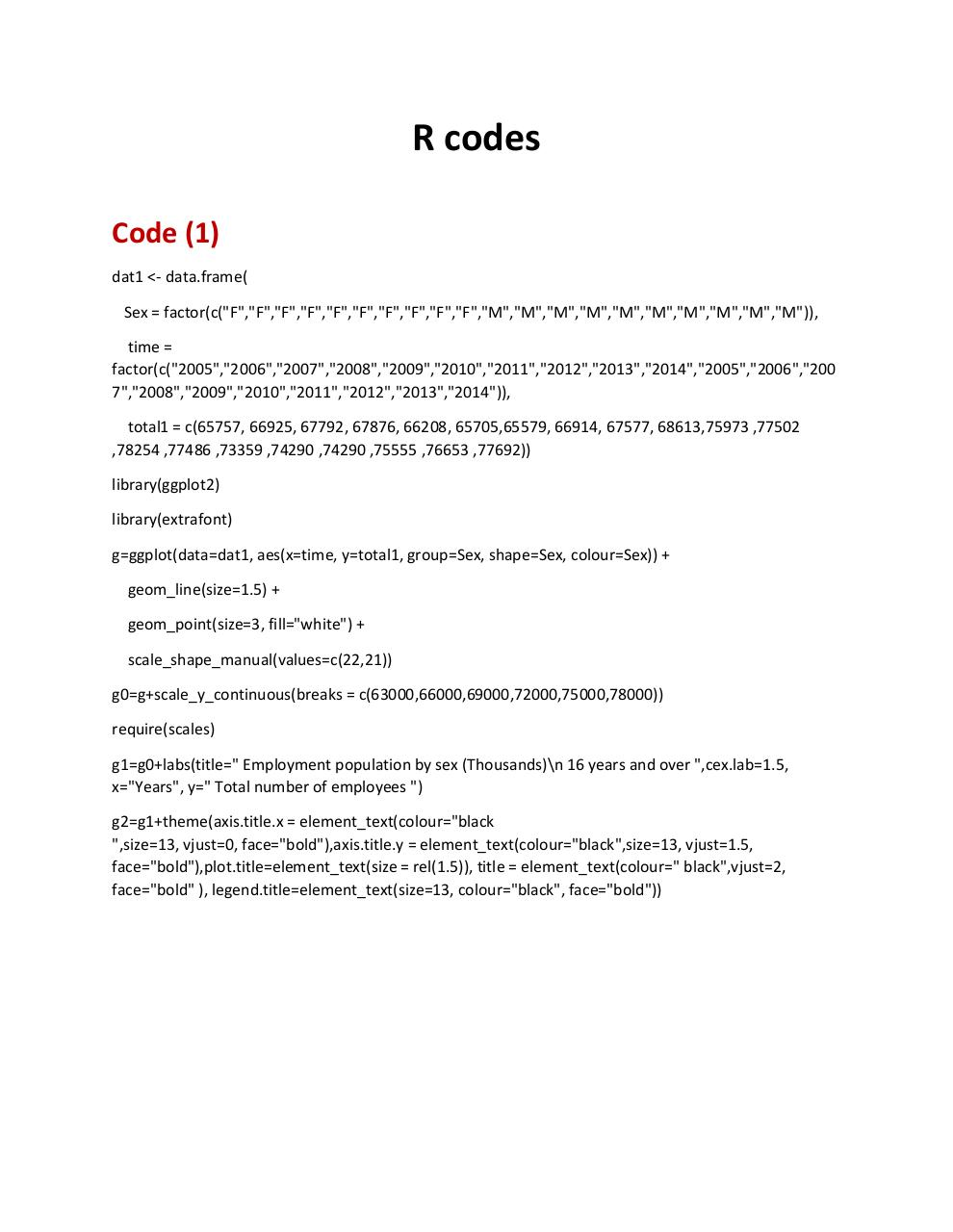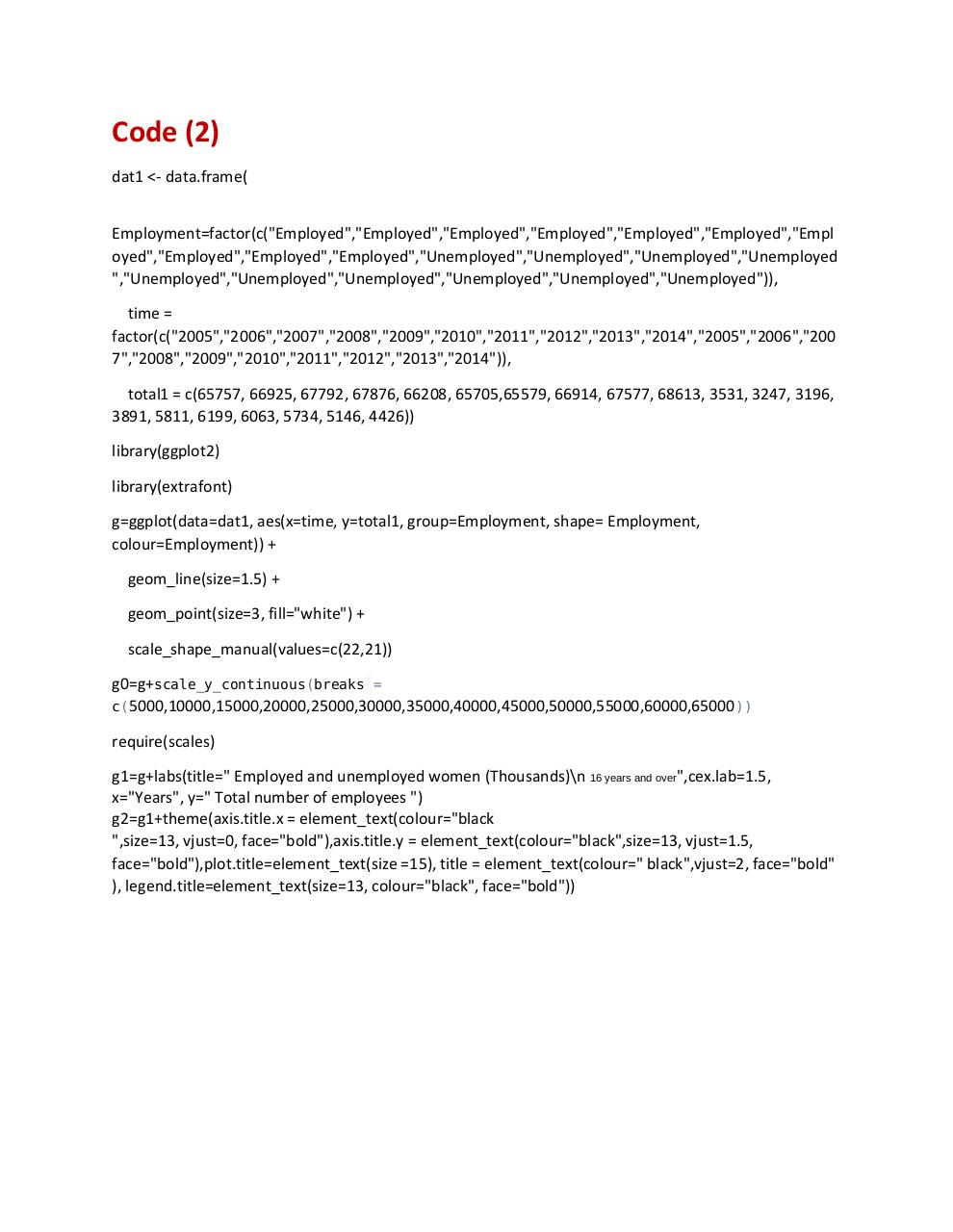# R Codes .pdf

### File information

Original filename: R Codes.pdf
Author: Owner

This PDF 1.5 document has been generated by MicrosoftÂ® Word 2013, and has been sent on pdf-archive.com on 31/10/2015 at 22:04, from IP address 129.174.x.x. The current document download page has been viewed 369 times.
File size: 196 KB (2 pages).
Privacy: public file

R Codes.pdf (PDF, 196 KB)

### Document preview

R codes
Code (1)
dat1 &lt;- data.frame(
Sex = factor(c("F","F","F","F","F","F","F","F","F","F","M","M","M","M","M","M","M","M","M","M")),
time =
factor(c("2005","2006","2007","2008","2009","2010","2011","2012","2013","2014","2005","2006","200
7","2008","2009","2010","2011","2012","2013","2014")),
total1 = c(65757, 66925, 67792, 67876, 66208, 65705,65579, 66914, 67577, 68613,75973 ,77502
,78254 ,77486 ,73359 ,74290 ,74290 ,75555 ,76653 ,77692))
library(ggplot2)
library(extrafont)
g=ggplot(data=dat1, aes(x=time, y=total1, group=Sex, shape=Sex, colour=Sex)) +
geom_line(size=1.5) +
geom_point(size=3, fill="white") +
scale_shape_manual(values=c(22,21))
g0=g+scale_y_continuous(breaks = c(63000,66000,69000,72000,75000,78000))
require(scales)
g1=g0+labs(title=" Employment population by sex (Thousands)\n 16 years and over ",cex.lab=1.5,
x="Years", y=" Total number of employees ")
g2=g1+theme(axis.title.x = element_text(colour="black
",size=13, vjust=0, face="bold"),axis.title.y = element_text(colour="black",size=13, vjust=1.5,
face="bold"),plot.title=element_text(size = rel(1.5)), title = element_text(colour=" black",vjust=2,
face="bold" ), legend.title=element_text(size=13, colour="black", face="bold"))

Code (2)
dat1 &lt;- data.frame(
Employment=factor(c("Employed","Employed","Employed","Employed","Employed","Employed","Empl
oyed","Employed","Employed","Employed","Unemployed","Unemployed","Unemployed","Unemployed
","Unemployed","Unemployed","Unemployed","Unemployed","Unemployed","Unemployed")),
time =
factor(c("2005","2006","2007","2008","2009","2010","2011","2012","2013","2014","2005","2006","200
7","2008","2009","2010","2011","2012","2013","2014")),
total1 = c(65757, 66925, 67792, 67876, 66208, 65705,65579, 66914, 67577, 68613, 3531, 3247, 3196,
3891, 5811, 6199, 6063, 5734, 5146, 4426))
library(ggplot2)
library(extrafont)
g=ggplot(data=dat1, aes(x=time, y=total1, group=Employment, shape= Employment,
colour=Employment)) +
geom_line(size=1.5) +
geom_point(size=3, fill="white") +
scale_shape_manual(values=c(22,21))
g0=g+scale_y_continuous(breaks =
c(5000,10000,15000,20000,25000,30000,35000,40000,45000,50000,55000,60000,65000 ))
require(scales)
g1=g+labs(title=" Employed and unemployed women (Thousands)\n 16 years and over",cex.lab=1.5,
x="Years", y=" Total number of employees ")
g2=g1+theme(axis.title.x = element_text(colour="black
",size=13, vjust=0, face="bold"),axis.title.y = element_text(colour="black",size=13, vjust=1.5,
face="bold"),plot.title=element_text(size =15), title = element_text(colour=" black",vjust=2, face="bold"
), legend.title=element_text(size=13, colour="black", face="bold"))#### HTML Code

Copy the following HTML code to share your document on a Website or Blog

#### QR Code### Related keywords